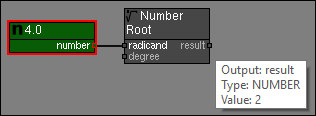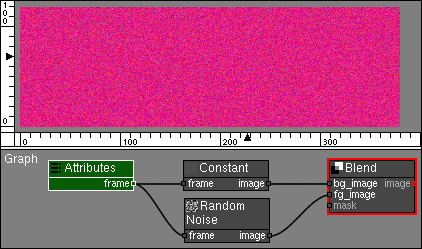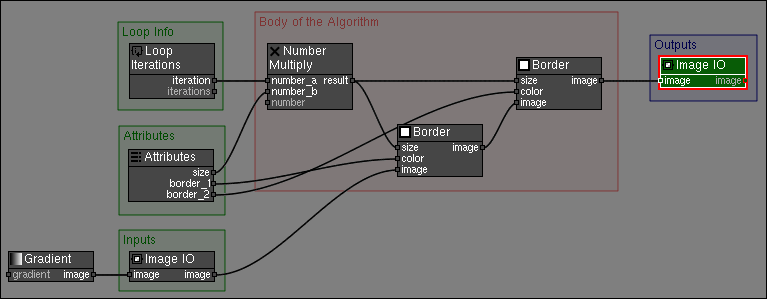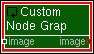# Visual Scripting with PixaFlux

Algorithm can be visualized as graphs where the operations are Nodes and the values flow between these nodes through Connections.

For example, the operation:

(6.0 ÷ 3.0) + (2.0 × 4.0)

can be visualized as this graph:Where:

• The number nodes [6.0] and [3.0] are connected as inputs of the division [÷] node.

• The number nodes [2.0] and [4.0] are connected as inputs of the multiplication [×] node.

• The results of the division and multiplication nodes are connected as inputs of the addition [+] node.

• The final result of the node graph is the result of the addition [+] node.

# PixaFlux

In PixaFlux this operation can be defined with nodes and connections:• The Number nodes define the values (6.0, 3.0, 2.0, 4.0).

• The Number Divide node divides the values returned as result from nodes [6.0] and [3.0].

• The Number Multiply node multiplies the values returned as result from nodes [2.0] and [4.0].

• The Number Add node adds the results from the Number Divide and Number Multiply nodes.

• The final result from the graph is returned by the result output of the Number Add node.

## Node Values

The Connection between two Nodes represents a value that flows from the output of one node to the input of other node.In the above image:

• The Number Node defines the number 4.0 and returns it as a NUMBER value in the output number.

• The Number Root Node receives as input the NUMBER value that was returned by the Number Node.

• The Number Root Node executes a square root operation on the input value and returns the result in it's result ouput.

PixaFlux supports the following types of Node Values:

• BOOLEAN. A boolean value. (true, false).
• NUMBER. A real number value. (0.0, 3.0, -2.0, 5.4, 3.141592).
• POINT. A 2D point or vector value ([0.0, 0.0], [1.0, -3.0], [2.4, 3.8]).
• FRAME. A rectangle defined by a position and size. (pos:[0.0, 0.0]; size:[120.0, 100.5]).
• RGBO. A red, greed, blue and opacity value. ().
• IMAGE. An image value.
• CURVES. A Bezier Splines container with multiple curves.
• TEXTURE. A procedural texture definition.

## Common Attributes

A Node in PixaFlux has Inputs, Outputs and Attributes. Inputs and Attributes can both be considered inputs of the operation that the node executes.

PixaFlux includes an Attributes Node that allows artists to create common attributes for the project, and node Attributes can be exposed as Inputs of the node.

This way two or more nodes can be affected by the same attribute in a consistent way:In this project: * The Attributes node defines a FRAME attribute which is returned by the node as the output frame. * The Constant node has the frame attribute exposed as input, and the Attributes node frame is connected to this input. * The Random Noise node has the frame attribute exposed as input, and the Attributes node frame is connected to this input. * The outputs of the Constant and Random Noise nodes are connected as inputs of the Blend node, which composes them over with a low opacity. * Editing the Attributes node frame attribute notifies the Constant and Random Noise nodes, which get the new FRAME from the Attributes node and update their image output. This way both nodes are guaranteed to always generate an image with the same frame, i.e. in the same position and with the same size.

This feature allows Attributes to be reused on the Node Graph and affect multiple nodes.

The Attributes node converts its attributes to Node Values. This is how they are converted:

Attribute Value Types to Node Value Types

• The BOOLEAN attribute value is converted to a BOOLEAN node value.
• The NUMBER attribute value is converted to a NUMBER node value.
• The POINT attribute value is converted to a POINT node value.
• The FRAME attribute value is converted to a FRAME node value.
• The RGBO attribute value is converted to a RGBO node value.
• The STRING attribute value is converted to a STRING node value.
• The CHOICE attribute value is converted to a STRING node value.

You can add to a PixaFlux project as Attributes nodes as you need, but the names of the attributes need to be unique across the project.

• Clock Tutorial

# Reusable Node Graphs

Node Graph projects are algorithms, and one of the main advantages of algorithms is that they can be reused.

PixaFlux projects can be saved to a PXF file and be reused in other projects using the Node Graph node.

### Elements of a Reusable Project

The main elements of a Reusable Project are:

Attributes

All attributes defined in Attributes nodes become common attributes of the project. Attributes nodes name private are ignored by the Node Graph node and can be used to define private attributes inside the project.

Inputs

The Inputs of the project can be defined with Input-Output (IO) nodes. PixaFlux looks for IO nodes with output connections and makes them input nodes.

Outputs

The Outputs of the project can be defined with Input-Output (IO) nodes. PixaFlux looks for IO nodes with no output connections and makes them output nodes.

Loop Info

The Loop Iterations node can be used in a reusable project to get the values of the number of iterations and the current iteration.When the Node Graph node loads a PixaFlux project it executes the following actions:

• The Node Graph node finds all the Attributes nodes in the pxf file and displays them in its attributes panel.

In the above example the size and two border color attributes are displayed in the Node Graph Node attributes panel.

• The Node Graph node finds all IO nodes that have no output connections and sets them as its outputs. The name of the output socket is set to the name of the IO node or the name of the output type if the name is not defined. If multiple input nodes have the same name, PixaFlux reports an error.

In this example the rightmost Image IO node has nothing connected to its output, so its set as an output of the Node Graph node.

• The Node Graph node finds all IO nodes with connections to their outputs and sets them as its inputs. Any nodes connected to the input of these nodes will be ignored by the Node Graph node. The name of the input socket is set to the name of the IO node or the name of the input type if the name is not defined. If multiple output nodes have the same name, PixaFlux reports an error.

In this example the Gradient node is ignored by the Node Graph node and the leftmost Image IO node is set as an input of the Node Graph node.

• The Node Graph node finds all Input and Output nodes of the same name and type and sets them as pairs. This enables the incremental modification of output values.

• IO nodes that have no connections are ignored by the Node Graph node.

• Node Frames are ignored by the Node Graph node.### Execution of Reusable Node Graphs

When the Node Graph node executes the project, it passes its inputs to the input IO nodes of the project and its attributes to the Attributes nodes of the project. If multiple iterations are executed, the Node Graph node passes the number of iterations and the current iteration to the Loop Iteration nodes of the node graph.

Then the node graph is executed the number of times defined in the Iterations attribute.

If the node graph includes pairs of input-output nodes, the values of the output nodes are copied to the input nodes.

Finally it copies the outputs of the IO nodes in the node graph to the outputs of the Node Graph node.

Main Page